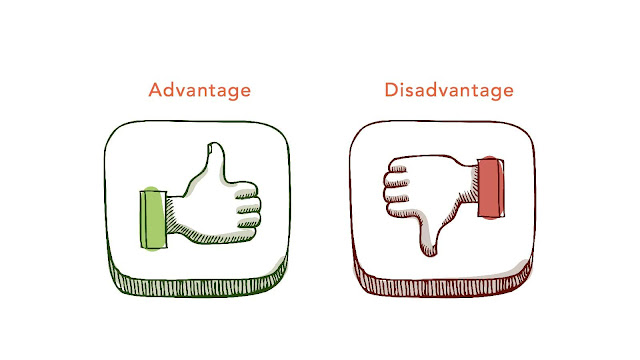# HSSlive: Plus One & Plus Two Notes & Solutions for Kerala State Board

## Thursday, January 26, 2023Advantages and Disadvantages of 2's Complement

## What is 2's complement

2's complement is a method used in digital logic and computer architecture to represent negative integers in binary form. It is a mathematical operation that is used to find the binary representation of the negative of a binary number. The 2's complement of a binary number is found by inverting all of the bits in the number and then adding 1 to the result. This method is commonly used in computer systems to represent negative integers and perform arithmetic operations on them. The advantage of using 2's complement is that it allows for efficient representation of negative numbers, and it also simplifies the process of adding and subtracting signed binary numbers. However, one disadvantage of using 2's complement is that it can lead to confusion and errors when working with large binary numbers, as the process of finding the 2's complement can be complex and error-prone. Additionally, 2's complement binary representation can lead to errors when converting to decimal representation.

2's complement is a method used in digital logic and computer architecture to represent negative integers in binary form. One of the main advantages of using 2's complement is that it allows for efficient representation of negative numbers. In most traditional methods of representing negative numbers, a separate sign bit is required to indicate whether a number is positive or negative. However, in 2's complement, negative numbers are represented using the same bit pattern as positive numbers, with the only difference being that the highest-order bit is used to indicate the sign.

Another advantage of 2's complement is that it simplifies the process of adding and subtracting signed binary numbers. Because 2's complement uses the same bit pattern for positive and negative numbers, the same hardware and algorithms can be used for both signed and unsigned arithmetic operations. This eliminates the need for separate hardware or software to handle signed and unsigned numbers, resulting in a more efficient and simpler system.

2's complement also provides a consistent and well-defined way of handling overflow and underflow conditions. It also makes it easy to convert between signed and unsigned numbers and vice-versa.

Additionally, 2's complement is widely used in computer systems and processors, making it a well-established and widely accepted method of representing negative numbers. This allows for easier interoperability between different systems and devices.

However, one disadvantage of 2's complement is that it can lead to confusion and errors when working with large binary numbers, as the process of finding the 2's complement can be complex and error-prone. Additionally, 2's complement binary representation can lead to errors when converting to decimal representation.

In conclusion, 2's complement is an efficient and widely accepted method of representing negative numbers in digital logic and computer architecture. Its advantages include efficient representation of negative numbers, simplification of signed binary operations, and consistency in handling overflow and underflow conditions. However, care must be taken to avoid confusion and errors when working with large binary numbers.

2's complement is a method of representing negative numbers in binary form. It is widely used in digital electronics and computer systems. One of the main advantages of 2's complement is that it simplifies the process of performing arithmetic operations on signed numbers. For example, when adding two numbers in 2's complement, the process is the same as when adding two unsigned numbers. This eliminates the need for separate logic circuits or software routines to handle signed numbers.

Another advantage of 2's complement is that it allows for simple overflow detection. When an overflow occurs during an arithmetic operation, the most significant bit of the result will be the opposite of the carry-out bit. This makes it easy to detect and handle overflow errors.

However, there are also some disadvantages of using 2's complement. One is that it can be more complex to understand and implement for beginners compared to other methods of representing negative numbers. Also, it can be more difficult to debug and troubleshoot errors in systems that use 2's complement, since the process of converting from 2's complement to decimal can be more complex. Additionally, it can be harder to detect some of the other errors such as underflow.

Another disadvantage is that it has a limited range of numbers that can be represented. It can only represent numbers in the range of -(2^(n-1)) to (2^(n-1))-1, where n is the number of bits used to represent the number. This can be a problem in systems that require a wider range of representable numbers.

In conclusion, 2's complement is a widely used method for representing negative numbers in binary form, with its main advantage being the simplification of arithmetic operations and overflow detection. However, it also has some disadvantages such as complexity, difficulty in debugging and a limited range of representable numbers.

Share: